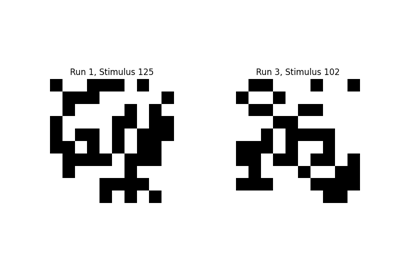Note

This page is a reference documentation. It only explains the function signature, and not how to use it. Please refer to the user guide for the big picture.

# 8.5.4. nilearn.image.coord_transform¶

nilearn.image.coord_transform(x, y, z, affine)[source]
Convert the x, y, z coordinates from one image space to another

space.

Parameters
xnumber or ndarray (any shape)

The x coordinates in the input space.

ynumber or ndarray (same shape as x)

The y coordinates in the input space.

znumber or ndarray

The z coordinates in the input space.

affine2D 4x4 ndarray

Affine that maps from input to output space.

Returns
xnumber or ndarray (same shape as input)

The x coordinates in the output space.

ynumber or ndarray (same shape as input)

The y coordinates in the output space.

znumber or ndarray (same shape as input)

The z coordinates in the output space.

Warning: The x, y and z have their output space (e.g. MNI) coordinate
ordering, not 3D numpy image ordering.

Examples

Transform data from coordinates to brain space. The “affine” matrix can be found as the “.affine” attribute of a nifti image, or using the “get_affine()” method for older nibabel installations:

```>>> from nilearn import datasets, image
## 8.5.4.1. Examples using `nilearn.image.coord_transform`¶